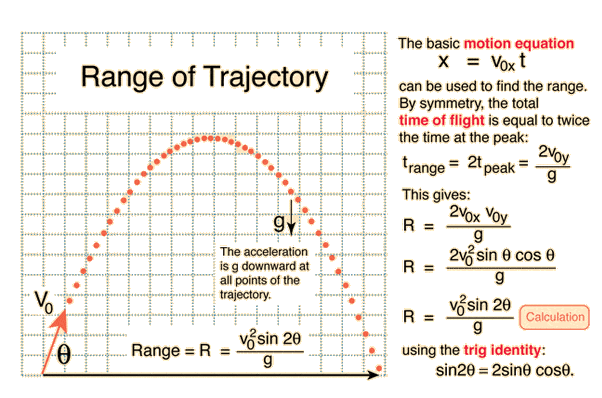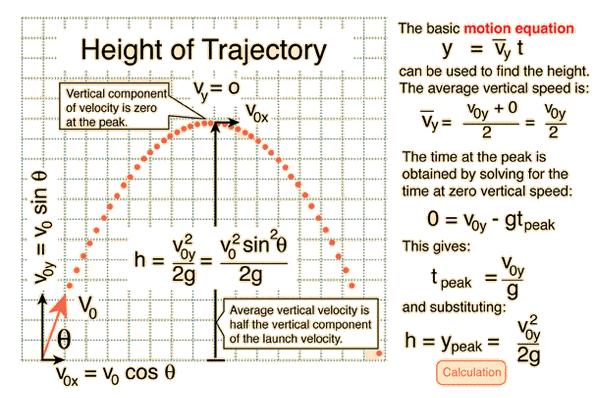# At what angle do you shoot a projectile so height=range?

And does the answer depend on gravity? In other words would it be the same angle on the moon?

berkeman
Mentor
And does the answer depend on gravity? In other words would it be the same angle on the moon?

What answers do you get when you do the math?

I got 0.5sin2x-sin(2x)=0 but I don't know how to algebraically solve that equation

berkeman
Mentor
I got 0.5sin2x-sin(2x)=0 but I don't know how to algebraically solve that equation

Can you show us the original kinematic equations that you started with and show each of your steps that got you to that equation?

SteamKing
Staff Emeritus
Homework Helper
I got 0.5sin2x-sin(2x)=0 but I don't know how to algebraically solve that equation
This is not an algebraic equation, so it can't be solved in that manner.

You can either plot it and find the solutions graphically or use a numerical technique, like iteration or Newton's method.

Since it is trigonometric, there are probably an infinite number of solutions, but you can restrict the range of angles which interest you.I used these equations. How do I solve the one I posted?

This is not an algebraic equation, so it can't be solved in that manner.

You can either plot it and find the solutions graphically or use a numerical technique, like iteration or Newton's method.

Since it is trigonometric, there are probably an infinite number of solutions, but you can restrict the range of angles which interest you.

O okay, so would Newton's method be the best numerical technique for this trigonometric equation that we can restrict the angles?

So I noticed that gravitational constant g cancels out of the equation, does that imply gravity is not a factor in answering this question? Which would imply that the same angle would work on the moon? Why is the answer not 45 degrees? Why is the answer greater?

Why is the answer 75.96? How is that intuitive? Why is it the same on every planet?

You can actually "solve" the equation. Use the formula sin(2Θ)=2sinΘ cosΘ.
One solution is of course Θ=0. Assuming Θ≠0 you can simplify sinΘ and reduce the equation to tanΘ=4.
Which is still a non algebraic equation but the solution can be found with a simple pocket calculator.

TY nasu. Why is the answer 75.96? Why is the answer not 45 degrees?

TY nasu. Why is the answer 75.96? Why is the answer not 45 degrees?
It's not 45 degrees for the same reason it's not 12, 38.987, or 756 degrees: it's not the right answer. Yes, there is some sort of similarity between the idea of equal height and distance, and the idea of an angle being equally far from vertical and horizontal, but you will find that picking answers to mathematical equations based on what seems sort of a right answer gives poor math grades and even more poorly designed bridges.

•billy_joule
Why is the answer 75.96? How is that intuitive? Why is it the same on every planet?
Who said is "intuitive"?
There is nothing special about this value. It is the value that satisfies that condition. If you ask for what angle the range is double the maximum height you will get another value for the angle. And so on.
For 45 degree the range has a maximum value (for the given value of speed) but is not equal to the maximum height.

Be careful, here "maximum" has slightly different meaning for the two quantities.
"Maximum" range assumes that you change the angle until the range has the largest value. For each one of this angle, the projectile will reach a "maximum" height on his trajectory. So there is a "maximum" height for each angle but the range is "maximum" only for 45 degrees.
This may be the source of your confusion. Or maybe my attempt to explain will be an even worse source of confusion. :)

256bits
Gold Member
oQUOTE="nasu, post: 4990279, member: 147320"]You can actually "solve" the equation. Use the formula sin(2Θ)=2sinΘ cosΘ.
One solution is of course Θ=0. Assuming Θ≠0 you can simplify sinΘ and reduce the equation to tanΘ=4.
Which is still a non algebraic equation but the solution can be found with a simple pocket calculator.[/QUOTE]
I would not have even used the equations of motion given, but deduced the answer from falling objects.
The projectile has to be aimed at a location P such that if an object is dropped from P and the projectile is fired at the same instance, they will both will fall for the same amount of time and also reach the ground at the same later instance.
At maximum height, h, the projectile will have travelled half the range, or a horizontal distance r. Knowing h = 2r, then P is at a height 4r. Thus simply by trigonometry, from the triangle with sides r and 4r, we can solve for the angle theta, and simply obtain, as you did, tanΘ=4r/r, or tanΘ=4.

Similar to "where do you aim if one wants to hit a target, if the shot is fired at the same time the target is dropped", which is a usual textbook exercise problem.

If it will save you some grief ive checked this out and the angle is fixed regardless of the value of g
its 75.96375.

A.T.